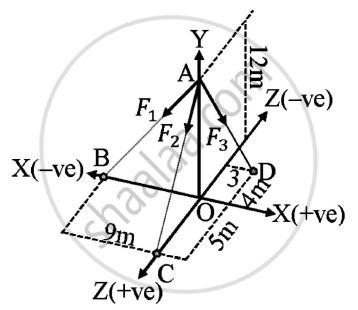# The Resultant of the Three Concurrent Space Forces at a is 𝑹̅ = (-788𝒋̅) N. Find the Magnitude of F1,F2 and F3 Forces. - Engineering Mechanics

The resultant of the three concurrent space forces at A is 𝑹̅ = (-788𝒋̅) N. Find the magnitude of F1,F2 and F3 forces.Given : A=(0,12,0)
B=(-9,0,0)
C=(0,0,5)
D=(3,0,-4)
Resultant of forces = (-788𝑗̅) N
To find : Magnitude of forces F1,F2,F3

#### Solution

Assume 𝑎̅,𝑏̅,𝑐̅ and 𝑑̅ be the position vectors of points A,B,C and D respectively w.r.t origin O
bar(OA) = 𝑎̅ = 12𝑗̅

bar(OB) = 𝑏̅ = -9𝑖̅
bar(OC)= 𝑐̅ = 5𝑘̅
bar(OD)= 𝑑̅ = 3𝑖̅ - 4𝑘̅

bar(AB)= 𝑏̅ - 𝑎̅
= -9𝑖̅-12𝑗̅
bar(AC) = 𝑐̅ – 𝑎̅ = 5𝑘 ̅- 12𝑗̅
bar(AD)= 𝑑̅ - 𝑎̅ = 3𝑖̅ -12𝑗 ̅ - 4𝑘

 Sr.no. Vector Magnitude 1. bar(AB) 15 2. bar(AC)  13 3. bar(AD) 13

 Sr.no. Vector "Unit vector "= ("vector")/("Magnitude of vector") 1. bar(AB) (−3)/5 bar(i) - 4/5 bar(j) 2. bar(AC)  (−12)/13 bar(j) - 5/13 bar(k) 3. bar(AD) (3)/13 bar(i) - 12/13 bar(j) - 4/13 bar(K)

Force along bar(AB) = bar(F1) = F1 ((−3)/5 bar(i) - 4/5 bar(j))

Force along bar(AC) = bar(F2) = F2((−12)/13 bar(j) - 5/13 bar(k))

Force along  bar(AB) = bar(F3) = F3( (3)/13 bar(i) - 12/13 bar(j) - 4/13 bar(K))

Resultant force bar(R) = bar(F1) +bar(F2)+ bar(F3)

-788 bar(j) = F1 ((−3)/5 bar(i) - 4/5 bar(j)+ bar(F2) (−12)/13 bar(j) - 5/13 bar(k) + bar(F3) (3)/13 bar(i) - 12/13 bar(j) - 4/13 bar(K))

0bar(j)-788 bar(j) + 0bar(k) = F1 ((−3)/5 bar(i) - 4/5 bar(j)+ bar(F2) (−12)/13 bar(j) - 5/13 bar(k) + bar(F3) (3)/13 bar(i) - 12/13 bar(j) - 4/13 bar(K))

Comparing the equation on both sides

(−3F1)/5 + (3F3)/13=0 ………..(1)
-(4F1)/5--(12F2)/13 -(12F2)/13=-788 ………(2)

(5F2)/13 -(4F3)/13 = 0  ……………(3)
Solving (1),(2) and (3)
F1=153.9063 N
F2=320.125 N
F3=400.1563 N

 Sr.no. Force Magnitude 1. F1 153.9063 N 2. F2 320.125 N 3. F3 400.1563 N
Concept: Resultant of concurrent forces
Is there an error in this question or solution?
2016-2017 (December) CBGS

Share